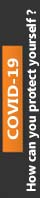Oct 16, 2017 term paper 2

# Explain the Difference Between Relative and Absolute Cell Referencing.

This paper concentrates on the primary theme of Explain the Difference Between Relative and Absolute Cell Referencing. in which you have to explain and evaluate its intricate aspects in detail. In addition to this, this paper has been reviewed and purchased by most of the students hence; it has been rated 4.8 points on the scale of 5 points. Besides, the price of this paper starts from £ 40. For more details and full access to the paper, please refer to the site.

After 36 months, what happens to a \$25,000 loan at 8% if the monthly payment is \$135?

1. This worksheet will compute the monthly value of an amortized loan for 36 months. This will be a worksheet in which the loan value or principal, interest rate, and monthly payment can be changed and the worksheet will automatically update. Within the worksheet there will be some absolute cell referencing that is needed and some relative cell referencing that is needed. You will need to determine which is appropriate.

a)Title the worksheet “Amortization of Car Loan” in cell A1. Leave a blank row under the title.

b)Enter Today’s Date in cell G1 so that it updates each time the file is updated in the spreadsheet.

2.In row five of this worksheet create cells labeled “Amount of Loan,”Interest Rate,” and “Monthly Payment,” with two columns between each label. Invent reasonable values to put in the three cells below each title as a starting place. These values are temporary values to help you see if your worksheet is working as you create it. For example, you may want to enter \$5000 as the loan amount, .065 as the interest rate, and \$150 as the monthly payment.

3.Leave two empty rows below the cells created in the prior step, then label four columns as “Month,”Current Principal,”Interest Due,” and “New Principal.”

• In cells under the “Month” title place the numbers from 1 to 36. Put the number 1 in the first cell and then use cell referencing and cell copying to create the rest of the column by using a formula that adds one to the cell above.
• In the first cell under “Current Principal” title reference the value in the “Amount of Loan” cell.
• In the first cell under the “Interest Due” column use a formula to calculate the interest that is due on the first value in the “Current Principal” column. You will need to multiply the “Current Principal” by the “Interest Rate,” divided by 12. (For the rate, reference the rate value entered in step 2. The rate is a yearly rate and the time is 1/12 of a year.) Use cell referencing in this formula. You will need to use an absolute reference to refer to the “Interest Rate” value. (The formula is I = p*r*t.)
• In the “New Principal” column use a formula to compute the new amount due by taking the “Current Principal” and adding the “Interest Due” and subtracting the “Monthly Payment.” Again, you must use absolute cell referencing for this.
• Make the next “Current Principal” equal to the “New Principal” from the month before.
• Complete the second row of the data by copying the rest of the formulas from the cells in the row above. (Check that your references are correct.)
• Complete the worksheet by copying all three of the formulas down for the remaining 36 months.
• At this point, you should be able to determine amounts due after 36 months by changing only the values of the “Amount of Loan,”Interest Rate,” and “Monthly Payment.” Test your worksheet by entering \$15000 into the “Amount of Loan,” 0.08 into the “Interest Rate,” and \$470 as the “Monthly Payment.” If you have completed everything correctly the amount due after 36 months is \$1.84! The interest due for the first month should be \$100.

4.Place the letters “a,”b,”c,” and “d” in a column to the right of the amortization work. Use your worksheet to determine the following and put the answer next to the letter:

a)After 36 months, how much will be owed on a loan of \$15,000 at 6% if the monthly payment is \$220?

b)Find the monthly payment to the nearest dollar (use trial and error and change the payment until you get it) that will pay off a \$123 ,000 loan in 36 months if the interest rate is 7%.

c)Find the monthly payment to the nearest dollar (use trial and error) that will pay off a \$7,000 loan in 36 months if the interest rate is 4%.

d)After 36 months, what happens to a \$25,000 loan at 8% if the monthly payment is \$135?

Explain this.

Explain the difference between relative and absolute cell referencing.

0% Plagiarism Guaranteed & Custom Written, Tailored to your instructions

International House, 12 Constance Street, London, United Kingdom,
E16 2DQ

## STILL NOT CONVINCED?

We've produced some samples of what you can expect from our Academic Writing Service - these are created by our writers to show you the kind of high-quality work you'll receive. Take a look for yourself!FLAT 25% OFF ON EVERY ORDER.Use "FLAT25" as your promo code during checkout